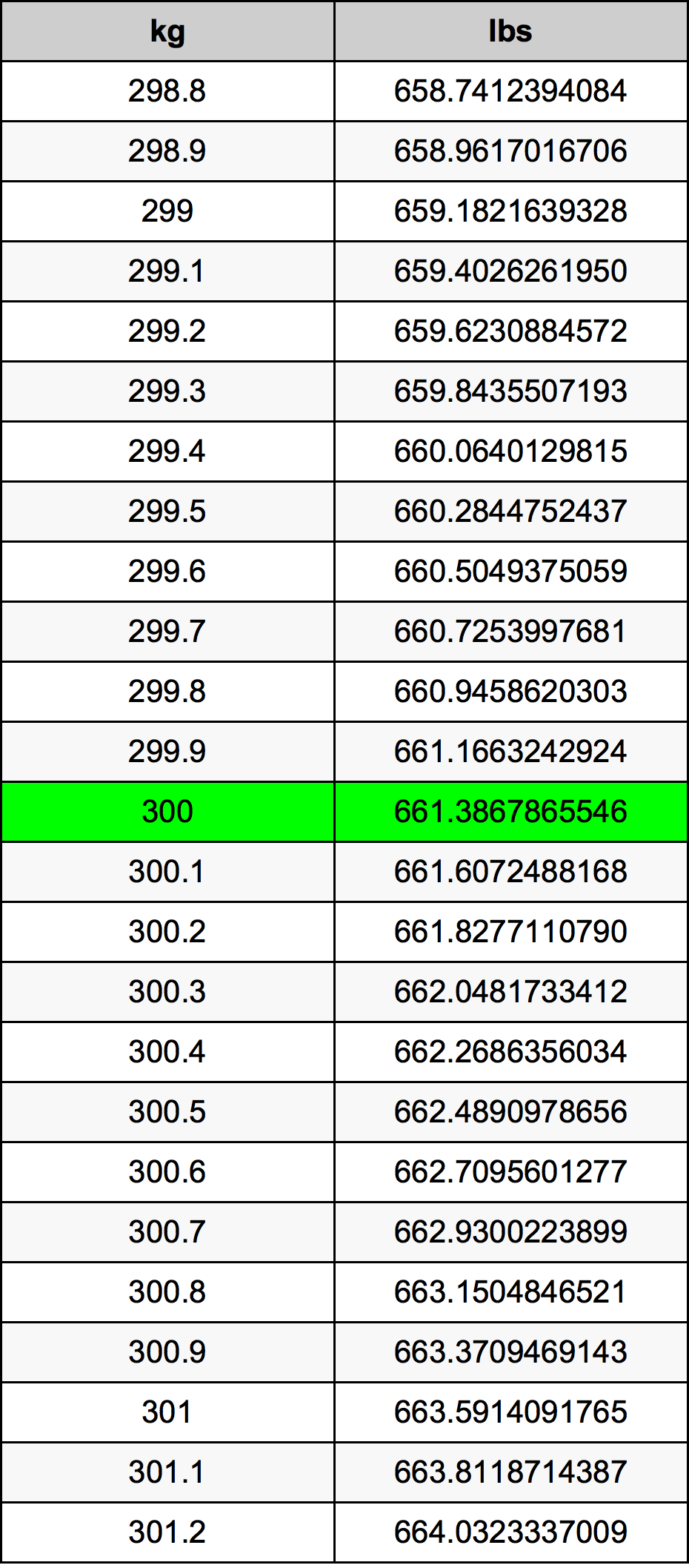Kg To Lbs

300 kg to lbs300 Kilograms to Pounds

kg
=
lbs

How to convert 300 kilograms to pounds?

 300 kg * 2.2046226218 lbs = 661.386786555 lbs 1 kg
A common question is How many kilogram in 300 pound? And the answer is 136.077711 kg in 300 lbs. Likewise the question how many pound in 300 kilogram has the answer of 661.386786555 lbs in 300 kg.

How much are 300 kilograms in pounds?

300 kilograms equal 661.386786555 pounds (300kg = 661.386786555lbs). Converting 300 kg to lb is easy. Simply use our calculator above, or apply the formula to change the length 300 kg to lbs.

Convert 300 kg to common mass

UnitMass
Microgram3e+11 µg
Milligram300000000.0 mg
Gram300000.0 g
Ounce10582.1885849 oz
Pound661.386786555 lbs
Kilogram300.0 kg
Stone47.2419133253 st
US ton0.3306933933 ton
Tonne0.3 t
Imperial ton0.2952619583 Long tons

What is 300 kilograms in lbs?

To convert 300 kg to lbs multiply the mass in kilograms by 2.2046226218. The 300 kg in lbs formula is [lb] = 300 * 2.2046226218. Thus, for 300 kilograms in pound we get 661.386786555 lbs.

300 Kilogram Conversion TableAlternative spelling

300 Kilograms to lbs, 300 Kilograms in lbs, 300 kg to Pounds, 300 kg in Pounds, 300 kg to Pound, 300 kg in Pound, 300 Kilogram to lb, 300 Kilogram in lb, 300 Kilogram to Pounds, 300 Kilogram in Pounds, 300 Kilogram to Pound, 300 Kilogram in Pound, 300 kg to lbs, 300 kg in lbs, 300 Kilograms to lb, 300 Kilograms in lb, 300 Kilogram to lbs, 300 Kilogram in lbs